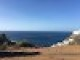(5 votes, average: 5.00 out of 5)Loading...

## Project Euler 112 Solution## Project Euler 112: Investigating the density of "bouncy" numbers.

#### Problem Description

Working from left-to-right if no digit is exceeded by the digit to its left it is called an increasing number; for example, 134468.

Similarly if no digit is exceeded by the digit to its right it is called a decreasing number; for example, 66420.

We shall call a positive integer that is neither increasing nor decreasing a "bouncy" number; for example, 155349.

Clearly there cannot be any bouncy numbers below one-hundred, but just over half of the numbers below one-thousand (525) are bouncy. In fact, the least number for which the proportion of bouncy numbers first reaches 50% is 538.

Surprisingly, bouncy numbers become more and more common and by the time we reach 21780 the proportion of bouncy numbers is equal to 90%.

Find the least number for which the proportion of bouncy numbers is exactly 99%.

#### Analysis

It was a surprise how long this program took to run. The is_bouncy() routine seemed to slow things down. We begin at the 90% point specified in the problem description and wait for the ratio to hit 99%.

#### Project Euler 112 Solution

Runs < 2.8 seconds in Python 2.7.Use this link to get the Project Euler 112 Solution Python 2.7 source.

#### Afterthoughts

Project Euler 112 Solution last updated

## Discussion

### One Response to “Project Euler 112 Solution”

1.A better way would be generate all the increasing(symmetrically decreasing) numbers. So the rest are bouncy numbers. No need to check all the numbers one by one

Posted by Yang Yang | March 29, 2011, 12:25 AM